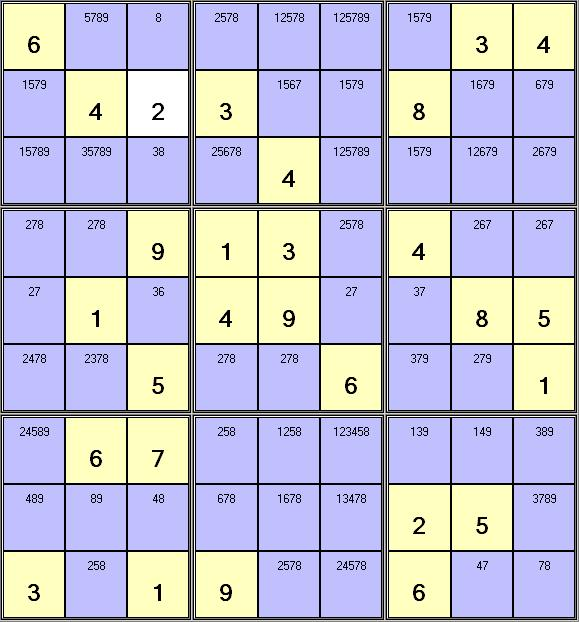# Sudoku of the Day - SolvingExample Puzzle with Notes

## Introduction

In the following explanations, when referring to the above example puzzle, an individual square is referred to as Cell(row, column), where rows and columns go from 1 to 9, starting in the upper left corner. For example, Cell(1,2) has the notes "5789".

## Singles

If you can reduce the number of possible values in any given cell to just one, then that is the value for that cell. In the above example puzzle, you can see that Cell(1,3) has been reduced to just the value "8" in the notes, so you can fill in 8 in that square.

## Hidden Singles

In any row/column or three by three box, if any number only occurs once in any of the lists of possible values, then that cell must take that value. Cell(5,3) is the only square that may contain a 6 in that column, so you can fill in 6 in that square.

## Naked Pairs

In any row/column or three by three box, if the same pair of possible values occurs twice, then those values can be eliminated as possibles from all the other cells. This rule can be extended easily to apply to naked triplets or quads. Cell(5,1) and Cell(5,6) form a naked pair of "27" for that row. That means you can eliminate the 7 from the notes in Cell(5,7). Cell(4,1), Cell(4,2) and Cell(5,1) form a naked triplet in that three by three box, so 2,7 and 8 can be removed from the notes of all the other squares in that box.

## Hidden Pairs

In any row/column or three by three box, if two numbers occur as possibles in only two cells, and they are the same cells, then all other numbers can be eliminated as possibles from those cells. This rule can be extended easily to apply to hidden triplets or quads. Cell(7,1) and Cell(9,2) are the only two squares that may contain a 2 or a 5 in that three by three box and therefore are a hidden pair. You can therefore eliminate the values 4,8 and 9 from the notes in Cell(7,1) and the 8 from Cell(9,2).

## Box/Row

Any number that has been eliminated as a possible from all of the cells in any two rows of a three by three box, must therefore occur in the remaining row of that box. It can therefore be eliminated as a possible from all of the cells in that remaining row in the other two three by three boxes. This rule can also be applied to columns.

## Row/Box

This is basically the same rule as "Box/Row" but being used in reverse. If any number has been eliminated from all the cells in any given row in two of the three by three boxes, then that number must be placed in the same row of the remaining box and can therefore be eliminated as a possible from the other two rows of the remaining box. This rule can also be applied to columns.Web www.sudokuoftheday.co.uk